How Cheenta works to ensure student success?
Explore the Back-Story

# NSEP 2015 Problem 9 | Pulley problem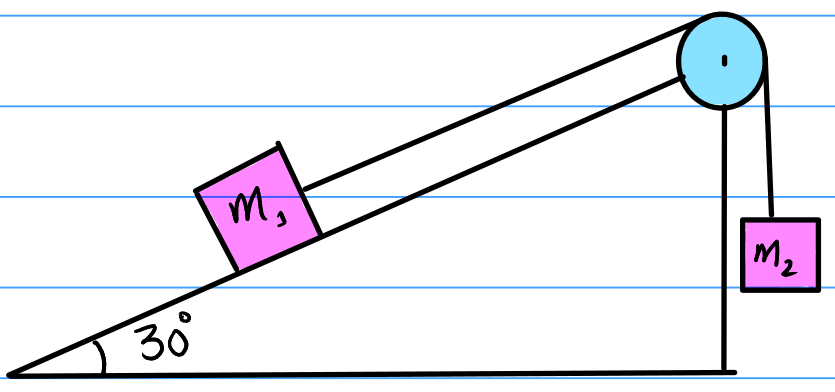Try this problem on pulley problem on inclined plane from NSEP 2015 Problem 9.

## NSEP 2015-16 ~ Problem 9

Maases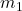andare connected to a string passing over a pulley as shown. Massstarts from rest and falls through a distance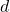in time t. Now, by interchanging the masses the time required forto fall through the same distance is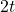. Therefore, the ratio of masses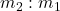a)b)c)d)### Key Concepts

Newton's Laws of Motion

Idea of accelerations, velocity and displacement

## Suggested Book | Source | Answer

Concept of Physics H.C. Verma

University Physics by H. D. Young and R.A. Freedman

Fundamental of Physics D. Halliday, J. Walker and R. Resnick

National Standard Examination in Physics(NSEP) 2015-2016

Option-(b)## Try with Hints

We know at the beginning the blocks have zero velocities. Using the relation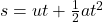, we can find the relation between the accelerations for two cases (i.e., when they are interchanged).

Knowing the accelerations we can now use the second law of newton to find the ratio of masses.

From the first hint,Now, we find the value of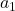andusing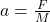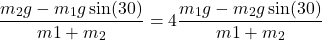Rearranging this expression and using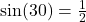,

This gives,## Subscribe to Cheenta at Youtube

Try this problem on pulley problem on inclined plane from NSEP 2015 Problem 9.

## NSEP 2015-16 ~ Problem 9

Maasesandare connected to a string passing over a pulley as shown. Massstarts from rest and falls through a distancein time t. Now, by interchanging the masses the time required forto fall through the same distance is. Therefore, the ratio of massesa)b)c)d)### Key Concepts

Newton's Laws of Motion

Idea of accelerations, velocity and displacement

## Suggested Book | Source | Answer

Concept of Physics H.C. Verma

University Physics by H. D. Young and R.A. Freedman

Fundamental of Physics D. Halliday, J. Walker and R. Resnick

National Standard Examination in Physics(NSEP) 2015-2016

Option-(b)## Try with Hints

We know at the beginning the blocks have zero velocities. Using the relation, we can find the relation between the accelerations for two cases (i.e., when they are interchanged).

Knowing the accelerations we can now use the second law of newton to find the ratio of masses.

From the first hint,Now, we find the value ofandusingRearranging this expression and using,

This gives,## Subscribe to Cheenta at Youtube

This site uses Akismet to reduce spam. Learn how your comment data is processed.

### Knowledge Partner